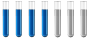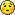## Calculation of standard molar enthalpy of formation

Chemistry and homework help forum.

Organic Chemistry, Analytical Chemistry, Biochemistry, Physical Chemistry, Computational Chemistry, Theoretical Chemistry, High School Chemistry, Colledge Chemistry and University Chemistry Forum.

Share your chemistry ideas, discuss chemical problems, ask for help with scientific chemistry questions, inspire others by your chemistry vision!

Please feel free to start a scientific chemistry discussion here!

Discuss chemistry homework problems with experts!

Ask for help with chemical questions and help others with your chemistry knowledge!

Moderators: expert, ChenBeier, Xen

anto007
Sr. MemberPosts: 32
Joined: Tue Aug 10, 2021 10:13 am
Location: Italy

### Calculation of standard molar enthalpy of formation

Hi everyone, I tried to do this exercise but I don't get the expected result from my text, would you help me understand where my mistake is? Thank you all.

The text of the exercise says: "If you burn at 25 ° C and 1 atm 0.100 dm ^ 3 of ethane, C2H6 (g), measured at 20 ° C and 1.50 atm you get 9.733 kJ of thermal energy. operates under the same conditions, for combustion of 0.284 g of carbon in the allotropic form of graphite 9.304 kJ of heat are obtained, while for combustion of 1,000 dm ^ 3 of hydrogen, measured at 18 ° C and 0.800 atm, 9.571 kJ are obtained. Calculate the molar enthalpy of ethane formation. "

First of all, I note that the heats reported in the text concern combustions conducted at 25 ° C and 1 atm so that the enthalpies that can be deduced are the standard ones, but I still calculate the combustion enthalpies anyway.

For C2H6 n=PV/RT = (1,50 atm* 0.100 L)/(0.08206 L atm mol^-1 K^-1 * 293.15 K)= 0.00623548 mol;
q=-9.733 kJ; ΔH°comb(C2H6)= -9.733 kJ/0.00623548= -1560.9 kJ mol^-1
For C n=0.284g/12.011g mol^-1= 0.023645 mol;
q=-9.304 kJ; ΔH°comb(C)= -9.304 kJ/0.023645 mol=-393.5 kJ mol^-1
For H n=PV/RT= (0.800 atm*1.000 L)/(0.08206 L atm mol^-1 K^-1*291.15 K)=0.0334843 mol
q=-9.571kJ; ΔH°comb(H)= -9.571kJ/0.0334843 mol= -285.8 kJ mol^-1

I apply Hess's law
4C(graphite)+ 4O2 --> 4CO2 ΔH°a= 4ΔH°comb(C)
6H2(g)+ 3O2(g) --> 6H2O (l) ΔH°b= 6ΔH°comb(H)
4CO2(g)+ 6H2O(l) --> 2C2H6(g)+ 7O2 ΔH°c= -2ΔH°com(C2H6)
____________________________________________________

4C(graphite)+ 6H2(g) --> 2C2H6 (g)
ΔH°f(C2H6)= [(-4 * 393.5)+(-6 * 285.8 )+(2 * 1560.9)]kJ mol^-1= -167 kJ mol^-1

but the result of my text is -84 kJ mol ^ -1, where did I go wrong?
The mind is like a parachute, it only works if it opens. -A. Einstein
ChenBeier
Distinguished MemberPosts: 916
Joined: Wed Sep 27, 2017 7:25 am
Location: Berlin, Germany

### Re: Calculation of standard molar enthalpy of formation

You calculate for 4C + 6H2 => 2 C2H6 -167 kJ/mol

But you can devide to 2C + 3H2 => C2H6 -83,55kJ/ mol ~ - 84 kJ/ mol
anto007
Sr. MemberPosts: 32
Joined: Tue Aug 10, 2021 10:13 am
Location: Italy

### Re: Calculation of standard molar enthalpy of formation

Right, what a fool I was!! Thanks so muchThe mind is like a parachute, it only works if it opens. -A. Einstein